# Writing the Equation of a Circle

Not only do you need to know how to graph a circle, but you also need to know how to write the equation of a circle when you see a graph.

To do this, you must once again remember the pattern for a circle:in which (h, k) is the center of the circle and r is the radius.

This time, however, you will follow these three steps:

1. Identify the center point and the radius from the graph.

2. Substitute that information back into the pattern

3. Simplify.

For example:

1. Find the equation of this circle: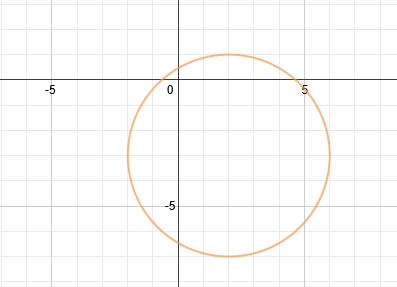The center point is not marked on this circle, but we can find it fairly easily:So from this we can tell that our center point is (2, -3) and the radius is 4. (To find the radius, simply count the number of spaces from the center point out to the circle.)

Now that we have our information, we will substitute it into the pattern:

Center: (2, -3) Radius: 4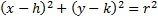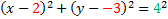Now we need to simplify:

Final answer: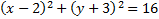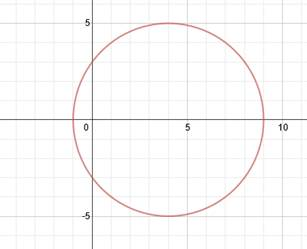Once again, let's mark the center point to make things more clear: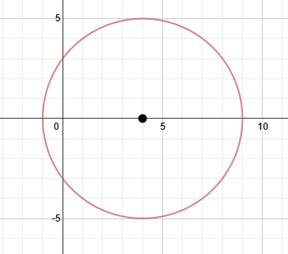The center point is (4, 0) and the radius is 5.

Now we'll substitute and simplify: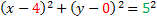Final answer: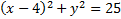Practice: Find the equation of these circles: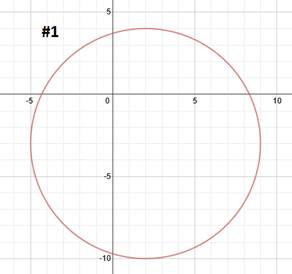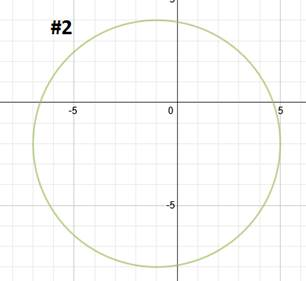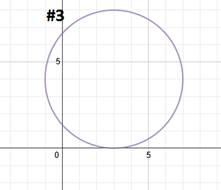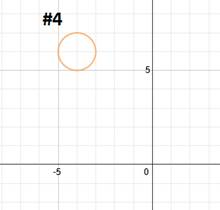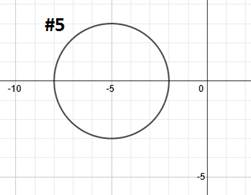Answers: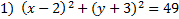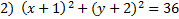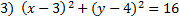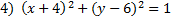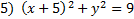Related Links: Math Fractions Factors